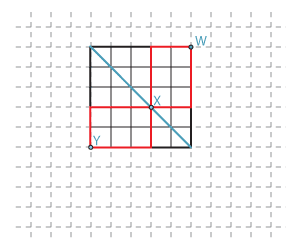## Answers to exercises

##### Exercise 1
1. There are $$3 \times 2 = 6$$ terms in the expansion.
2. There are $$2^5 = 32$$ terms in the expansion.
##### Exercise 2

$$c_2 =\dbinom{12}{2} = 66, \quad c_3 =\dbinom{12}{3} = 220, \quad c_5 =\dbinom{12}{5} = 792, \quad c_9 =\dbinom{12}{9} = 220$$.

##### Exercise 3

The possible strings of $$R$$'s and $$U$$'s have length $$m+n$$. If the grid has height $$m$$ and width $$n$$, then there must be $$m$$ $$U$$'s and $$n$$ $$R$$'s. So the number of ways of choosing the paths is $$\dbinom{m+n}{n} = \dbinom{m+n}{m}$$.

##### Exercise 4

The binomial theorem gives

$(1+x)^n = \dbinom{n}{0}+ \dbinom{n}{1}x + \dbinom{n}{2}x^2+\dots+\dbinom{n}{r}x^r+\dots+ \dbinom{n}{n-1}x^{n-1} + \dbinom{n}{n}x^n.$
1. When $$x=2$$, we have
\begin{align*} 3^n&=(1+2)^n \\ &= \dbinom{n}{0}+ \dbinom{n}{1}2 + \dbinom{n}{2}2^2+\dots+\dbinom{n}{r}2^r+\dots+ \dbinom{n}{n-1}2^{n-1} + \dbinom{n}{n}2^n\\ &=1+ 2\dbinom{n}{1}+ 4\dbinom{n}{2}+\dots+2^r\dbinom{n}{r}+\dots+ 2^{n-1}\dbinom{n}{n-1} + 2^n. \end{align*}
2. When $$x=-2$$, we have
\begin{align*} &(-1)^n =(1-2)^n \\ &= \dbinom{n}{0}+ \dbinom{n}{1}(-2) + \dbinom{n}{2}(-2)^2+\dots+\dbinom{n}{r}(-2)^r+\dots+ \dbinom{n}{n-1}(-2)^{n-1} + \dbinom{n}{n}(-2)^n\\ &=1 - 2\dbinom{n}{1}+ 4\dbinom{n}{2}+\dots+(-2)^r\dbinom{n}{r}+\dots+ (-2)^{n-1}\dbinom{n}{n-1} + (-2)^n. \end{align*}
##### Exercise 5
1. $$(2x-3y)^4 = 16x^4 - 96x^3y + 216x^2y^2 - 216xy^3 + 81y^4$$
2. $$\Big(x-\dfrac{2}{x}\Big)^4 =24 + \dfrac{16}{x^4} - \dfrac{32}{x^2} - 8 x^2 +x^4$$
##### Exercise 6

There are eight terms in the expansion.

The general term is $$(-2)^r\dbinom{7}{r}a^{7-r}b^{3r}$$, for $$0\leq r\leq 7$$.

##### Exercise 7

The constant term is 15.

##### Exercise 8

The middle term is $$-4320 x^3 y^3$$.

##### Exercise 9

$$c_9=2^6\times3^9\dbinom{15}{9}=6\ 304\ 858\ 560$$

##### Exercise 10

In the following diagram, there are $$\tbinom{3+2}{3}$$ paths from $$Y$$ to $$X$$, and $$\tbinom{3+2}{2}$$ paths from $$X$$ to $$W$$. So the number of paths from $$Y$$ to $$W$$ that pass through $$X$$ is

$\dbinom{3+2}{3} \dbinom{3+2}{2} =\dbinom{5}{3} \dbinom{5}{2} = \dbinom{5}{2}^2.$

Every path from $$Y$$ to $$W$$ meets the diagonal, and so the total number of paths from $$Y$$ to $$W$$ can be found by summing the number of such paths through each point on the diagonal. The general result follows from the same argument.Detailed description of diagram

##### Exercise 11

Assume that the coefficients of three consecutive powers of $$(1+x)^n$$ are in arithmetic progression. Let the three coefficients be

$\dbinom{n}{r-1}, \qquad \dbinom{n}{r}, \qquad \dbinom{n}{r+1}.$

Then

\begin{align*} \dbinom{n}{r} -\dbinom{n}{r-1} &= \dbinom{n}{r+1} -\dbinom{n}{r}\\ \dfrac{n!}{(n-r)!r!} - \dfrac{n!}{(n-r+1)!(r- 1)!} &= \dfrac{n!}{(n-r -1)!(r+1)!} - \dfrac{n!}{(n-r)!r!}\\ \dfrac{n!}{(n-r)!(r-1)!}\Bigg(\dfrac{1}{r}-\dfrac{1}{n-r+1}\Bigg) &= \dfrac{n!}{(n-r-1)!r!}\Bigg(\dfrac{1}{r+1}-\dfrac{1}{n-r}\Bigg)\\ \dfrac{n-2r+1}{n-r+1}&=\dfrac{n-2r-1}{r+1}\\ (n-2r)^2&=n+2. \end{align*}

For this to be possible, $$n+2$$ is a perfect square.